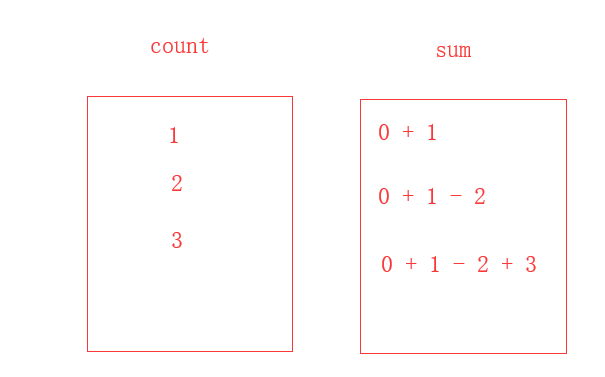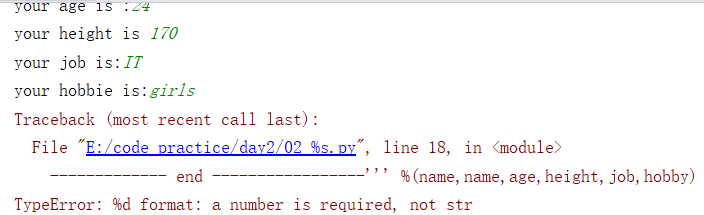# day02 格式化输出和初始编码

### 1. day 01 内容回顾

#### （3）如何终止循环？

1.改变条件，使其不成立；
2.使用break 或者 continue 语句

### 2. day01 homework答案参考

#### （1）使用while循环输入 1 2 3 4 5 6 8 9 10

# 第一：加入空格这个思路
number = 0
while number < 10:
number += 1
if number == 7:
print(' ')
else:
print(number)

#第二：Pass 什么都不执行
number = 0
while number < 10:
number += 1
if number == 7:
pass
else:
print(number)

# 第三，最优解，最简洁
number = 0
while number < 10:
number += 1
if number == 7:
continue
print(number)


#### （3）（4）输出1-100所有的奇数

# 第一种：直接让count自加2即可，count += 2
count = 1
while count < 101:
print(count)
count += 2

# 第二种：标准写法，奇数对2取余数为1，然后打印这些数即是奇数。
count = 1
while count < 101:
if count % 2 == 1:
print(count)
count += 1


#### （5）求1-2+3-4+5 …+99的所有数的和count = 1
sum = 0
while count < 100:
if count % 2 == 1:
sum = sum + count
if count % 2 == 0:
sum = sum - count
count += 1
print (sum)


#### （6）用户登陆（三次机会重试）

tries = 0
while tries < 3:
print ('登陆成功')
else:
print('登录失败，请重新登录')
tries += 1
else:
print('输错超过三次，您的用户已经被锁定，请20分钟后再试')


### 3. %格式化输出

name = input('请输入你的姓名：')
age = input('请输入你的年龄：')
height = input('请输入你的身高：')
print ('我的名字是：',name '我的年龄是：', age '我的身高是：',height)
#输出



------------ info of Alex Li -----------
Name  : Alex Li
Age   : 22
job   : Teacher
Hobbie: girl
------------- end -----------------


**格式化输出：**其实就是占位，告诉计算机这个地方我后面需要根据用户的输入，来进行替换

name = input('your name is :')
age = input('your age is :')
height = input('your height is ')
info = '''------------ info of %s ----------- # 每一个%s 相当于一个占位符，替换name
Name  : %s  #代表nane
Age   : %d  #代表age
Height: %d  #代表height
job   : %s  #代表job
Hobby: %s  #代表hobby
------------- end -----------------''' %(name,name,age,height,job,hobby)
print(info)info = "我是%s,年龄%d,目前学习进度为3%"%('Ric',18)
print(info)


info = "我是%s,年龄%d,目前学习进度为3%%"%('Ric',18)
print(info)


info = "我是%s,年龄%d,目前学习进度为3%%s"%('Ric',18)
print(info)
#输出



### 4. 初始编码

00000 代表“你” 0101 代表 “好” 0100 代表“啊”。但是，把它们放在一起，就不知到是什么意思了。
0000001010100 计算机在不知道以上含义的前提下能准确识别吗？

8bit = 1 byte （一个字节）
1024 byte = 1 KB
1024 KB = 1 MB
1024 MB =1 GB
1024 GB = 1TB

Unicode

Utf-8
UTF-8，是对Unicode编码的压缩和优化，他不再使用最少使用2个字节，而是将所有的字符和符号进行分类：

gbk：中国国产，只用于中文和ascii码中文字。用一个字节表示英文，两个字节表示中文

### 5. 运算符

#### 逻辑运算符

print(3>4 or 4<3 and 1==1) # False
print(1 < 2 and 3 < 4 or 1>2) # True
print(2 > 1 and 3 < 4 or 4 > 5 and 2 < 1) #True
print(1 > 2 and 3 < 4 or 4 > 5 and 2 > 1 or 9 < 8) # False
print(1 > 1 and 3 < 4 or 4 > 5 and 2 > 1 and 9 > 8 or 7 < 6) #False
print(not 2 > 1 and 3 < 4 or 4 > 5 and 2 > 1 and 9 > 8 or 7 < 6) #False


print(0 or 2) #2
print(3 or 2) #3
print(1 and 2) #2
print(0 and 2) #0


（1）x or y , x为真，值就是x，x为假，值是y；

（2）x and y, x为真，值是y,x为假，值是x

print(1 > 2 and 3 or 4 and 3 < 2)


1.False and 3 or 4 and False
2.False or False

print(2 or 1 < 3) # 2
print(3 and 1 < 3) #True
print(0 or 1 < 3)  #True
print(0 and 1 < 3) #0
print(5 and 3 < 1 and 2) #False
print(0 or 3 < 1 and 2) #False


Q：python中的>或者<之类的运算符的优先级比or大，比and小？

05-291048
12-13551
10-26
09-21
09-16
09-18
09-21
02-05
09-17

### “相关推荐”对你有帮助么？

•非常没帮助
•没帮助
•一般
•有帮助
•非常有帮助被折叠的  条评论 为什么被折叠?到【灌水乐园】发言Lircsome

¥2 ¥4 ¥6 ¥10 ¥20余额支付 (余额：-- )扫码支付获取中扫码支付点击重新获取扫码支付1.余额是钱包充值的虚拟货币，按照1:1的比例进行支付金额的抵扣。
2.余额无法直接购买下载，可以购买VIP、C币套餐、付费专栏及课程。余额充值